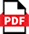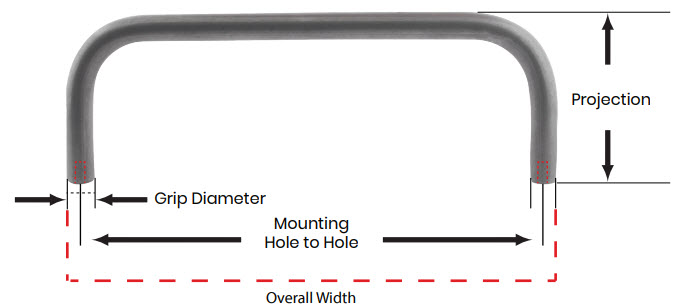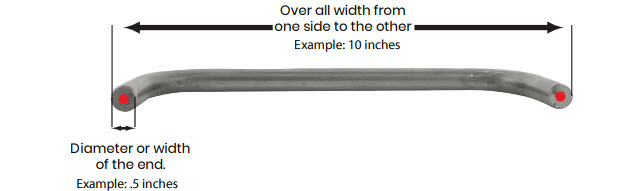## Pull Handles - Threaded Holes MeasurementWhen asked to measure “on center” it refers to measureing from the center of one screw hole to the center of the other screw hole. There are two dimensions that you need to determine where the mounting point to mounting point, center hole to center hole is for mounting a pull handle.1. You need to determin the overall width. You simply measure one side to the other on the outside of the part. For example, let’s say it’s 10 inches.2. You need the measurement of the diameter or width of the end. For example, in this case it would be .5 inches. If you want to get to the center to center measurement all you do is subtract half the diameter from each side. That would be .25 inch from each side. You can add the two halfs together which is .5 inch.

Finally take the overall width which was 10 inches (in our example) and subtract what the two half’s of the end diameter, .25 + .25 = .5 inch and subtract. 10 - .5 = 9.5. There for your center to center measurement for the threaded hole pull handle is 9.5 inches.

Updated:10/22/18